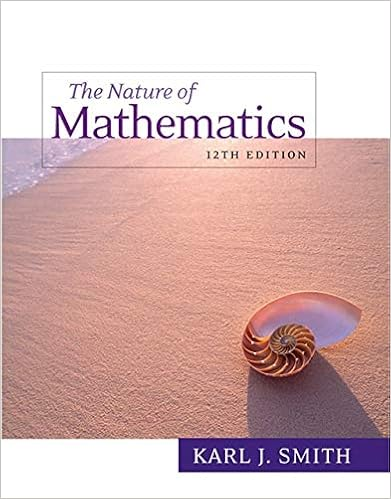# Construct a stem and leaf plot of the following data

• Test Prep
• nrfry
• 12

This preview shows page 2 - 6 out of 12 pages.

##### We have textbook solutions for you!
The document you are viewing contains questions related to this textbook.The document you are viewing contains questions related to this textbook.
Chapter 12 / Exercise 23
Nature of Mathematics
SmithExpert Verified
4.Construct a Stem and Leaf Plot of the following data:1.4,2.3,2.1,4.0,3.6,1.2,2.5,4.5,1.2,2.5,3.1,3.7,4.0,4.0,5.1,4.2,5.32
##### We have textbook solutions for you!
The document you are viewing contains questions related to this textbook.The document you are viewing contains questions related to this textbook.
Chapter 12 / Exercise 23
Nature of Mathematics
SmithExpert Verified
5.Consider the following list of 24 data points:18301515232610712292516191620411182022271730a.Complete the following table:Class BoundaryClassFrequencyCumulative FrequencyClass Midpoint1-6b.Construct a histogram for the data in the above table.3
6.There are four lab sections of a class and the number of students in each section is listedin the following table:SectionNumber of StudentsSection 116Section 284Section 314Section 495a.Find the total number of students enrolled in this class and calculate the percentageof students in each section.b.In order to test the students satisfaction of the Minitab manual, sections 1 and 3were randomly selected and all of their 30 students were interviewed. Does thissampling plan result in a systematic, cluster, or stratified sample? Explain.7.Consider the following sample of data values:37,42,28,23,19,32,40,24,33,29,20,22,25,31,33The corresponding sample mean and standard deviation arex= 29.2ands= 7.10a.Find thez-scores of 19 and 42 and determine whether they are usual or unusual.b.Find the percentile of the data value 31.4
c.FindP77.d.FindP40.8.A spinner wheel contains 8 regions numbered 1 through 8.The arrow has an equallylikely chance of landing on any of the 8 regions.a.Find the probability that the arrow lands on an odd number.b.If the spinner wheel is spun twice, find the probability that the arrow lands first onan odd number, then on a 5.9.A box contains 5 blue marbles, 13 red marbles, and 7 white marbles.a.Find the probability that a randomly selected marble is blue.b.Find the probability of selecting first a blue marble and secondly a white marble ifthe selection is done without replacementwithout replacementwithout replacement.c.
•••